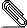# [HACKERS] Hash Join cost estimates

```All,

Marty's issue w/ NestLoop reminded me that I'd put together a test
case which illustrates a HashJoin doing the wrong thing.```
```
The test case is here:

http://snowman.net/~sfrost/test_case.sql

Simply load that up and then check out this plan:

explain select * from small_table join big_table using (id_short);

We end up deciding to build a hash table of 4M records and then seq
scan the table that only has 41K.  Much of the reason for this is
because the analytics point out, correctly, that the column we're
using from the small table to join isn't unique and therefore the
buckets will be deeper- but it's not *nearly* as bad as we estimate.

The bucket estimate for the small table comes back as 26, while the
reality is that we only look through ~5.5 entries per bucket with
the longest run being 21.  With the big table being hashed, the bucket
estimate is 4 (though this seem to vary way more than I would expect,
sometimes 4, sometimes 8, sometimes 2..) while the average number
scanned through is actually ~3.5 and the longest scan ends up being
20.

Without the bucket question, we end up with pretty reasonable results
(directly out of initial_cost_hashjoin):

41K hashed, seqscan 4M:
startup_cost = 1229.46
run_cost = 72307.39

4M hashed, 41K seqscan:
startup_cost = 115030.10
run_cost = 817.70

When we get through dealing with the bucketing question in
final_cost_hashjoin (costsize.c:2673), we have some pretty gross
results for the 'good' plan's run_cost:

run_cost = 72307.39 + 138848.81 = 211156.20

While the 'bad' plan's run_cost is only bumped a tiny bit:

run_cost = 817.7 + 411.76 = 1229.46

Resulting in total costs that look all kinds of wacky:

41K hashed, seqscan 4M: 115030.10 + 1229.46 = 116259.56
4M hashed, seqscan 41K: 1229.46 + 211156.20 = 212385.66

Or the 'good' plan being costed at *nearly double*.  Now, my laptop
might not be the 'best' system CPU wise, but there's a pretty massive
time difference between these plans:

41K hashed, seqscan 4M: 2252.007 ms http://explain.depesz.com/s/FEq
4M hashed, seqscan 41K: 2708.471 ms http://explain.depesz.com/s/FOU

That's half a second and a good 15+% difference.

Now, back to the bucket estimation- the ndistinct for the small table
is -0.475058 (or 19561 tuples), which is about right.  There are 23
distinct values, 18,795 duplicated, and another 841 with dup counts
ranging from 3 to 10.  This leads to an avgfreq of 0.000051,
unfortunately, we're going for only 8192 buckets, so this gets moved
up to 0.00012 and then the adjustment for MCV (which is 0.00027) bumps
this all the way up to 0.00064, leading to our bucket depth estimate
of 26 (bucket size estimate multiplied by the inner rows) and the
resulting cost dominating the overall costing.

If we consider the bucketing wrong and look at what the costs would
have been with the actual number of average scans (and therefore
excluding the 0.5 'adjustment'), we get:

seqscan 41K cost: 360.28 (cpu_op_cost * 41K * 3.5)
seqscan 4M cost: 58743.73 (cpu_op_cost * 4M * 5.5)

which isn't exactly going in the 'right' direction for us.  Now, I'm
sure that there's a cost to having to consider more buckets, but it
seems to be far less, in comparison to the hash table creation cost,
than what our model would suggest.

In the end, I think the problem here is that we are charging far too
much for these bucket costs (particularly when we're getting them so
far wrong) and not nearly enough for the cost of building the hash
table in the first place.

Thoughts?  Ideas about how we can 'fix' this?  Have others run into
similar issues?

Thanks,

Stephen
```signature.asc
Description: Digital signature